# 字符串模式匹配KMP算法详解（Python语言）

##### 问题描述

主串为${}^{\prime }ababcabcacba{b}^{\prime }$$'ababcabcacbab'$，模式串为${}^{\prime }abca{c}^{\prime }$$'abcac'$，现在要求模式串在主串中出现的位置。

##### 暴力解法

直接用两层循环，从主串的第一个位置和模式串的第一个位置开始，依次比较字符是否相等，如果相等，则继续比较下一个；如果不相等，则从主串的第二个位置重新和模式串的字符匹配。完整匹配过程如下图所示：（图片截图与电子书，数组下标从$i=1$$i=1$开始，和程序中有些不同，读者注意一下）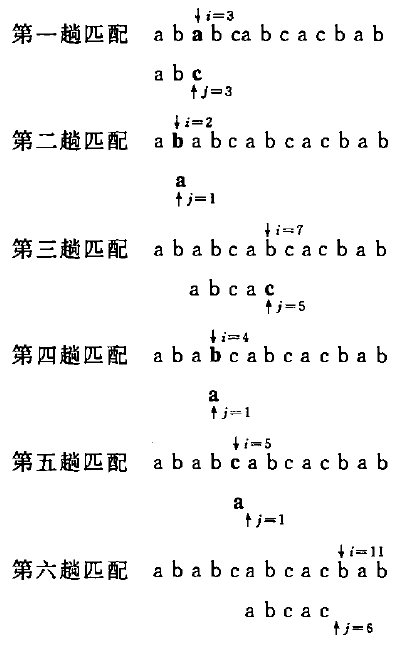暴力解法的代码如下，很简单：

def index(self, S, T):
"""
:type S: str
:type T: str
:rtype: int
"""
i = 0
j = 0
while i < len(S) and j < len(T):
if S[i] == T[j]:    # 依次比较，相等则比较下一个字符
i += 1
j += 1
else:    # 如果不相等，指针i需要回溯到上个起点的下一个位置
# 并从头开始比较
i -= j - 1
j = 0
# while循环结束后，要么是找到合适匹配了，要么是遍历完主串都没有找到合适匹配
if j == len(T):
return i - j
else:
return -1

如果主串长度为$n$$n$，模式串长度为$m$$m$，那么暴力法最坏的时间复杂度为$O\left(m\ast n\right)$$O(m*n)$。但在一般情况下，其实际的执行时间近似于$O\left(m+n\right)$$O(m+n)$，因此这个方法至今仍被采用。

##### KMP算法

分析一下暴力法的匹配过程，每次重新开始匹配模式串，我们都需要从模式串的第一个位置重新开始，如果主串中有很多和模式串“部分匹配”的情况，这种方法就显得很累赘了，其实有很多比较过程都可以跳过的。改进的过程如下图所示：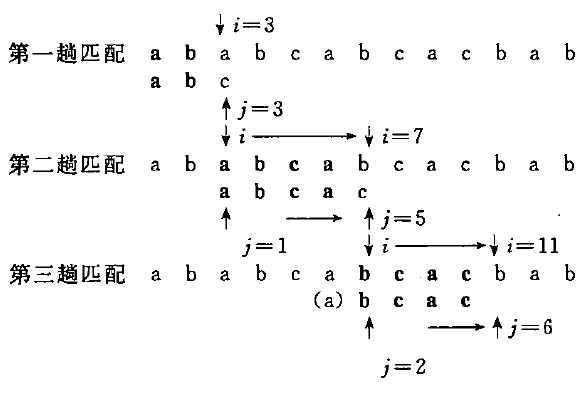这就是著名的KMP算法，它最大的特点就是主串的指针$i$$i$不需要回溯！不需要回溯！不需要回溯！重要的事情说三遍。整个匹配过程中，对主串仅需从头到尾扫描一遍即可，这对处理从外设输入的庞大文件很有效，可以边读边匹配，无序回头重读。那么KMP算法是如何做到这一点的呢？可能上面这个例子特殊性还不够，我们现在对原问题进行稍稍一点变化：主串改为${}^{\prime }acabaabaabcacaab{c}^{\prime }$$'acabaabaabcacaabc'$，模式串为${}^{\prime }abaabca{c}^{\prime }$$'abaabcac'$，它的匹配过程如下图所示：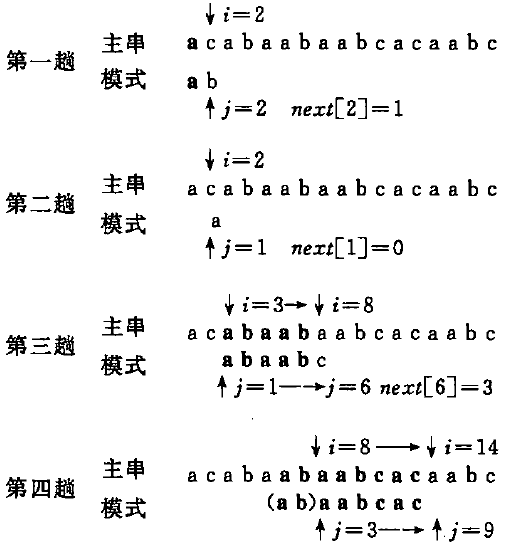现在重点关注第三趟匹配过程，前面5个字符${}^{\prime }abaa{b}^{\prime }$$'abaab'$匹配成功，在第6个字符时$a\ne c$$a \ne c$匹配失败。我们注意到${}^{\prime }abaa{b}^{\prime }$$'abaab'$前两个字符和后两个字符一样，那么我们是不是就可以跳过前两个字符，直接从模式串的第三个字符开始比较？这就是KMP算法的核心所在。只要我们在之前匹配成功的模式串中发现这种“首尾相等”的情况，那么我们下一次可以直接跳过首尾相等的这一部分子串（如上图中第四趟括号中的字符）；当然如果第一个字符就匹配失败，那就还是用暴力法。所以KMP算法仅当模式与主串之间存在许多“部分匹配”的情况下才比暴力法快得多。
现在讨论一般情况，假设主串为${s}_{1}{s}_{2}\dots {s}_{n}$$s_1s_2 \dots s_n$，模式串为${p}_{1}{p}_{2}\dots {p}_{m}$$p_1p_2\dots p_m$，本轮匹配在${s}_{i}\ne {p}_{j}$$s_i \ne p_j$处失败。我们需要考虑这样一个问题，${s}_{i}$$s_i$下一次应该与模式串中的哪个字符比较？假设此时应与模式中第$k$$k$个字符${p}_{k}$$p_k$$k$k）继续比较，我们记$next\left[j\right]=k$$next[j]=k$，它表示模式中第$j$$j$个字符${p}_{j}$$p_j$与主串字符${s}_{i}$$s_i$匹配失败时，模式中重新和${s}_{i}$$s_i$进行比较字符的位置（这个定义很重要！时刻记着！）。那么${s}_{i}$$s_i$的前$k-1$$k-1$个字符一定与模式串中${p}_{k}$$p_k$$k-1$$k-1$个字符相等（比如第四趟中${s}_{6}{s}_{7}={p}_{1}{p}_{2}$$s_6s_7=p_1p_2$），即

$\begin{array}{}\text{(1)}& {s}_{i-k+1}{s}_{i-k+2}\dots {s}_{i-1}={p}_{1}{p}_{2}\dots {p}_{k-1}\end{array}$

$\begin{array}{}\text{(2)}& {s}_{i-k+1}{s}_{i-k+2}\dots {s}_{i-1}={p}_{j-k+1}{p}_{j-k+2}\dots {p}_{j-1}\end{array}$

$\begin{array}{}\text{(3)}& {p}_{1}{p}_{2}\dots {p}_{k-1}={p}_{j-k+1}{p}_{j-k+2}\dots {p}_{j-1}\end{array}$

我自己看到这里时有个疑问：为什么能保证${s}_{4}$$s_4$${s}_{7}$$s_7$这些位置不可能发生正确匹配？现在假设这个算法漏了${s}_{5}$$s_5$这个位置，也不存在什么“首尾相等”的情况（$next\left[j\right]$$next[j]$找到的一定是首尾相等的情况），上一轮匹配情况是${s}_{3}{s}_{4}{s}_{5}{s}_{6}{s}_{7}={p}_{1}{p}_{2}{p}_{3}{p}_{4}{p}_{5}$$s_3s_4s_5s_6s_7=p_1p_2p_3p_4p_5$${s}_{8}\ne {p}_{6}$$s_8 \ne p_6$，如果${s}_{5}$$s_5$是一个答案，就至少要满足${p}_{1}{p}_{2}{p}_{3}={s}_{5}{s}_{6}{s}_{7}={p}_{3}{p}_{4}{p}_{5}$$p_1p_2p_3=s_5s_6s_7=p_3p_4p_5$，然后这不又是首尾相等了？这和假设是矛盾的，假设不成立。
现在来看看KMP基本的算法流程，假设现在我们已经求得了正确的$next$$next$函数（这个KMP中最难理解的一个地方，目前暂且将它视为一个黑盒子）。在进行模式匹配过程中，每次遇到${s}_{i}\ne {p}_{{j}_{1}}$$s_i \ne p_{j_1}$的情况，就调用$next\left[{j}_{1}\right]$$next[j_1]$函数得到${s}_{i}$$s_i$下一个要比较的${p}_{{j}_{2}}$$p_{j_2}$，如果${p}_{k}={s}_{i}$$p_k=s_i$则继续向下比较，否则继续用$next\left[{j}_{2}\right]$$next[j_2]$找到下一个${j}_{3}$$j_3$，如果$next$$next$找不到下一个位置了，则说明最终主串和模式串匹配成功的那部分子串中不可能包含${s}_{i}$$s_i$这个位置的字符，所以这时我们就需要放弃${s}_{i}$$s_i$${s}_{i+1}$$s_{i+1}$处从头和模式串进行匹配。代码如下，重申一下，书中下标是从1开始的，程序中是从0开始的，所以会稍有不同：

def kmp(self, S, T):
i = 0
j = 0
while i < len(S) and j < len(T):
if j == -1 or S[i] == T[j]:    # 当匹配成功时，往下继续匹配
# 当j=-1时，表示找不到下一个点，从s[i+1]开始重新和T匹配
i += 1
j += 1
else:    # 匹配不成功，用next(j)找到下一个比较起点
j = next(j)    # 如果找不到下一个点，返回-1
# while循环结束后，要么是找到合适匹配了，要么是遍历完主串都没有找到合适匹配
if j == len(T):
return i - j
else:
return -1

这么看KMP是不是还比较简单，和暴力法相比，就是多了一个$next$$next$函数。错！！！就是它花了我一下午时间！！！接下来我们来讲讲怎么实现这个$next$$next$函数。（又回到下标为1的背景，懒得自己作图，见谅哈哈）
直觉上我们只要找到上一次匹配成功的那部分模式串中首尾相等的那部分子串，然后移动模式串让首尾对齐即可，$k-1$$k-1$就是相等子串的长度。$next\left[j\right]$$next[j]$和主串无关，函数值仅取决于模式串本身，可以递推出下列模式串$next$$next$函数值：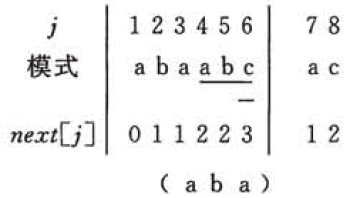乍一看，这不是就是用模式串匹配它自身吗？这和上面岂不是一样？NoNoNo，还是有点差别的。我们约定，当$next\left[j\right]$$next[j]$不存在时，返回0（程序中是-1，数组起始下标不同的原因），即图中第二趟匹配所示：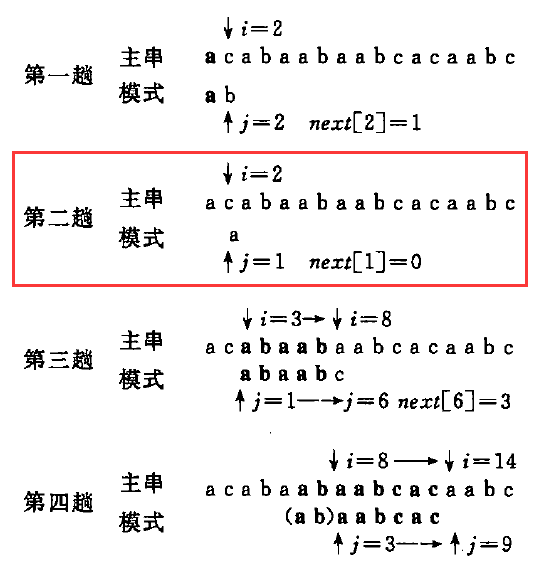第二趟中因为${s}_{2}$$s_2$${p}_{1}$$p_1$直接就不匹配了，${p}_{1}$$p_1$前面已经没有前缀，当然也不存在接下来什么“首尾相等”的情况，所以就返回0这个没有实际意义的数字。然后下一轮直接从${s}_{3}$$s_3$开始重头匹配。所以$next\left[1\right]=0$$next=0$第一项就确定了，接下来用$next\left[j\right]$$next[j]$来递推$next\left[j+1\right]$$next[j+1]$（不要说用暴力法找相同前缀后缀，不然KMP也就失去了时间效率上面的优势）。
现在假设$next$$next$数据前$j$$j$项已经全部知道了，且$next\left[j\right]=k$$next[j]=k$，这说明${p}_{1}\dots {p}_{j-1}$$p_1\dots p_{j-1}$$k-1$$k-1$项和后$k-1$$k-1$项相等：

${p}_{1}\dots {p}_{k-1}={p}_{j-k+1}\dots {p}_{j-1}$
那现在如果加入一个${p}_{j}$$p_j$，那么对于新得到的${p}_{1}\dots {p}_{j}$$p_1\dots p_{j}$$next\left[j+1\right]$$next[j+1]$会等于多少呢？这里分两种情况来讨论：
(1) 当${p}_{j}={p}_{k}$$p_j=p_k$时，就相当于前缀和后缀各增加一个相同的字符，可以接起来，所以$next\left[j+1\right]=k+1$$next[j+1]=k+1$
(2) 如果${p}_{j}\ne {p}_{k}$$p_j\ne p_k$，那不就接不起来了？怎么办？举个例子说明一下（用Excel截的图，将就看一下）。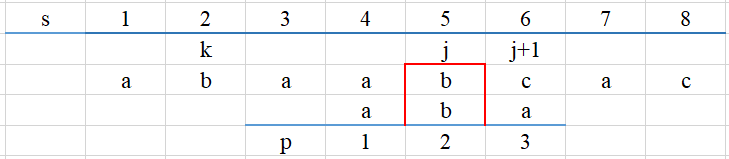现在比方说$j=5$$j=5$，那么$k=next\left[5\right]=2$$k=next=2$（假设$next\left[1\right]\sim next\left[5\right]$$next \sim next$都是已知的），所以下一步应当比较${s}_{5}$$s_5$${p}_{2}$$p_2$。因为${s}_{5}={p}_{2}$$s_5=p_2$，所以相当于前缀后缀各增加一个字符$b$$b$，所以$next\left[6\right]=next\left[5\right]+1=3$$next=next+1=3$，这对应着第(1)种${p}_{j}={p}_{k}$$p_j=p_k$的情况。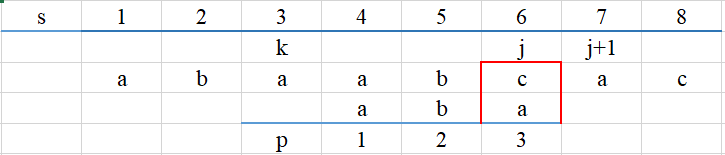现在我们来计算$next\left[7\right]$$next$。我们已经知道$k=next\left[6\right]=3$$k=next=3$，所以用${p}_{3}$$p_3$来和${s}_{6}$$s_6$比较，但我们发现${p}_{3}\ne {s}_{6}$$p_3 \ne s_6$$next\left[7\right]$$next$就是要求${s}_{7}$$s_7$下一步应该和模式串中的那个字符比较，才能使${s}_{7}$$s_7$前面的前缀$j\le 6$$j \le 6$尽可能长，当前$abc\ne aba$$abc \ne aba$，所以需要继续右移模式串，寻找符合这个要求的位置。根据KMP的思想，这个右移操作岂不是可以用$next\left[3\right]=1$$next=1$来表示？于是比较${p}_{6}=={p}_{1}$$p_6==p_1$，但不幸的是，又不相等，只好用$next\left[1\right]=0$$next=0$继续寻找点，发现不存在，找不到${s}_{7}$$s_7$得前缀，只好从头开始匹配，所以$next\left[7\right]=1$$next=1$。纵览整个过程，不匹配的情况就是不断利用$next$$next$前面几轮的信息在模式串的不匹配字符位前面的子串中跳跃地寻找匹配点的过程。$next$$next$的代码如下（分析了一大堆，代码结果只有一丢丢，好气啊）：

def get_next(self, T):
i = 0    # 指向主串的指针
j = -1   # 指向模式串的指针，一开始
next_val = [-1] * len(T)    # 要返回的next数组
while i < len(T)-1:    # next=-1,只需要求后面的m-1个值即可
if j == -1 or T[i] == T[j]:    # 匹配成功，相同前缀长度增加1；找不到时直接开始下一位
i += 1
j += 1
next_val[i] = j
else:    # 匹配不成功则在前面的子串中继续搜索，直至找不到
j = next_val[j]
return next_val

但是上面的$next$$next$函数还是有一丢丢缺陷，例如模式$aaaab$$aaaab$，上面得到的结果是$-1,0,1,2,3$$-1,0, 1, 2, 3$，这意味着前面重复的$a$$a$还是进行了比较，但是明明可以一口气跳过前面的重复部分，直接比较第4个$a$$a$，我们可以通过在上面的函数中加一个小小的条件判断进行改进：

def get_next(self, T):
i = 0    # 指向主串的指针
j = -1   # 指向模式串的指针，一开始
next_val = [-1] * len(T)    # 要返回的next数组
while i < len(T)-1:    # next=-1,只需要求后面的m-1个值即可
if j == -1 or T[i] == T[j]:    # 匹配成功，相同前缀长度增加1；找不到时直接开始下一位
i += 1
j += 1
if i < len(T) and T[i] != T[j]:
next_val[i] = j
else:     # 如果字符重复则跳过
next_val[i] = next_val[j]
else:    # 匹配不成功则在前面的子串中继续搜索，直至找不到
j = next_val[j]
return next_val

终于把$next$$next$这个大头搞定了，最后看下完整的KMP算法：

class Solution:
# 获取next数组
def get_next(self, T):
i = 0
j = -1
next_val = [-1] * len(T)
while i < len(T)-1:
if j == -1 or T[i] == T[j]:
i += 1
j += 1
# next_val[i] = j
if i < len(T) and T[i] != T[j]:
next_val[i] = j
else:
next_val[i] = next_val[j]
else:
j = next_val[j]
return next_val

# KMP算法
def kmp(self, S, T):
i = 0
j = 0
next = self.get_next(T)
while i < len(S) and j < len(T):
if j == -1 or S[i] == T[j]:
i += 1
j += 1
else:
j = next[j]
if j == len(T):
return i - j
else:
return -1

if __name__ == '__main__':
haystack = 'acabaabaabcacaabc'
needle = 'abaabcac'

s = Solution()
print(s.kmp(haystack, needle))    # 输出 "5"